Electricity And Energy Class 10th Science Tamilnadu Board Solution

Question 1.Veena’s car radio will run from a 12 V car battery that produces a current of 0.20 A even when the car engine is turned off. The car battery will no longer operate when it has lost 1.2 x 106 J of energy. If Veena gets out of the car, leaving the radio on by mistake, how long will it take for the car battery to go completely dead, i.e. lose all energy?(1 day = 86400 second)Answer:In this question we need to find the time taken (T) by the car to go dead when the radio is left on by Veena , We are given with the amount of energy car can supply (E) which is 1.2 X 106 .We are also given with the car battery’s potential difference (V) which it can give which is 12 V , and the amount of current produced (I) is 0.2 A .By using the equation , E = V× I× T ,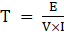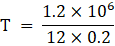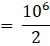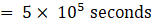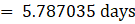1 day = 86400 seconds, 5 X 105 seconds =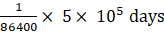Question 2.Find the total current that passes through the circuit. Find the heat generated across the each resistor.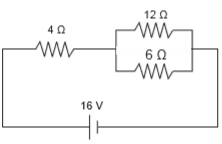Answer:First we need to find the total current (I) flowing in the circuit, that we can find by using Ohm’s law, because we are given with the battery’s potential difference (V) and we need to find the net resistance of the circuit (R).As we can see there are 3 resistors 4 ohm, 6 ohm and 12 ohm, and there are two resistors in parallel the 6 and 12 ohm ones, sowe will first solve the parallel ones and get the parallel resistance Rp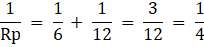Rp = 4 ohmNow the net resistance will come by simply adding the Rp = 4 ohm and the other 4 ohm resistor because they both are now in series,So,R = Rp + 4 = 4 + 4 = 8 ohmR = 8 ohmBy using Ohm’s law, V = I× R,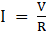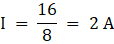Now we need to find the heat generated across each resistor, which we will do by using Joule’s law of heating,Mathematically,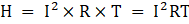Where I is current passing through conductor; T is time; R is resistance of the conductor; and H is heat generated across the conductor.As the 4 ohm resistor is alone and in series so current I1 passing through it will be equal to the total current passing through the circuit ,Which is 2 A and the potential difference across the 4 ohm resistor isV = I× R = 2× 4 = 8 VNow the two resistors 6 and 12 ohm are in parallel that is they have same potential difference across them which is 8 V because the battery has total potential difference of 16 V and 8 V is consumed by the 4 ohm resistor which is in series and alone so , 16-8 = 8 V the remaining potential difference is 8 V which is consumed by both 6 and 12 ohm resistors in parallel.Let the current passing through 6-ohm resistor be I2 and current passing through 12 ohm resistor be I3 .By Ohm’s law,V = I2× R2,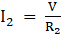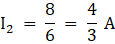V = I3× R3 ,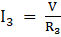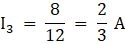Heat generated by 4-ohm resistor,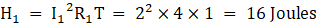Heat generated by 6-ohm resistor,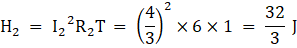Heat generated by 12-ohm resistor,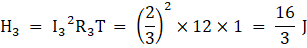Time will be same for all the resistors always, in this case as no time is given so we will calculate for one second of heat generated or we can leave the answer in terms of T.Question 3.Find the total current that passes through the circuit given in the diagram. Also find the potential difference across 1Ω resistor.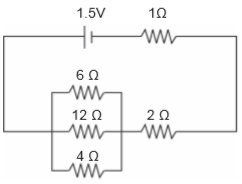Answer:To find the total current(I) passing through the circuit, we must find the total resistance(R) and also we know the battery’s potential difference(V) which is 1.5 V , so by Ohm’s law we can find the net current flowing in the circuit.As we can see by the figure that two resistors 1 ohm and 2 ohm are in series to each other, so we can directly add them , 1 + 2 = 3 ohm,Also we can see that 3 resistors 4 ohm , 6 ohm , and 12 ohm are parallel to each other , so , let the net parallel resistance of these three resistors be Rp ,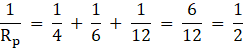Therefore, Rp = 2 ohmHence by Ohm’s law, V = I× R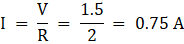As the current passing through 1 ohm resistor is the net current flowing in the circuit, soV = I× R = 0.75× 1 = 0.75 V. It is the potential difference(V) across the 1 ohm resistor.Question 4.Raman’s air-conditioner consumes 2160 W of power, when a current of 9.0 A passes through it.i) What is the voltage drop when the air-conditioner is running?ii) How does this compare to the usual household voltage?iii) What would happen if Raman tried connecting his air-conditioner to a 120V line?Answer:We are given the amount of power consumed by Raman’s air-conditioner when a current of 9 A flows through it.(i). The meaning of voltage drop simply means the potential difference across Raman’s air conditioner when it is running which we have to find ,Now to find the potential difference by knowing power and amount of electric current passing through passing through it we can use this relation ,P = V× I ,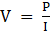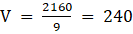V(ii). In India, we have a household voltage of 220 V current supply,which changes time to time and in very short time intervals.As there is no major difference in 220 V and 240 V A.C. supply , which we will study in higher classes not in this class in detail , So yes these are comparable voltages and this air conditioner can be used in houses.(iii). If raman tries to connect his air conditioner to a 120 V supply line , then his air conditioner would do less amount of work and deliver less power(rate of doing work) , also as it’s potential difference is reduced to half and of course it’s resistance is constant , so the current passing through it would also become less and will be reduced to half itself , so basically the power would become 1/4th of the original power , also this situation might destroy your appliance or the air conditioner in Raman’s case.Question 5.The effective resistance of three resistors connected in parallel is 60/47 Ω. When one wire breaks, the effective resistance becomes 15/8 ohms. Find the resistance of the wire that is broken.Answer:As the three resistors are in parallel combination to each other , so , let the total resistance of the 3 resistors be R3 and the total resistance of the two resistors be R2 ,Let the three resistors be Ra , Rb , Rc .First we will calculate the total resistance of 3 resistors,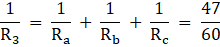We are given with the values of R3 = 60/47 ohm and R2 = 15/8 ohms ,When one wire is broken down , let the wire be of resistance Ra , so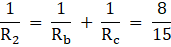Put the value of two resistor equation in three resistor equation ,We get ,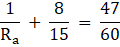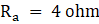Hence this is the required resistance.Question 6.Find the resistance acrossA and D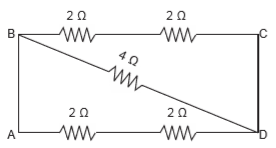Answer:In order to find the total resistance, first we need to memorize the rule which we have learned which is , THE POINTS WHO’S ACROSS WE HAVE TO EVALUATE THE TOTAL RESISTANCE ARE THE GIVEN POINTS AND WE CONSIDER OR IMAGINE THAT A BATTERY IS CONNECTED TO THE CIRCUIT THROUGH THESE TWO POINTS ONLY .And the rest are the normal points of the circuit.A and D are the given points in this part of the question and the rest of the points are the simple points (that is B and C).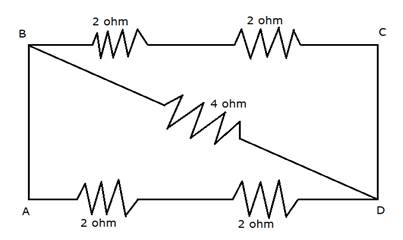As the resistors in the line BC are in series and the resistors in the line AD are also in series with each other , so they will get added like integers or they will be simply added.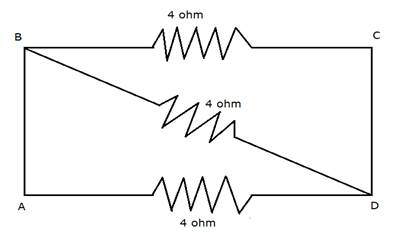Now merge the points B into A and C into D , because they have same potential , all the three resistors are parallel to each other ,By solving we will get ,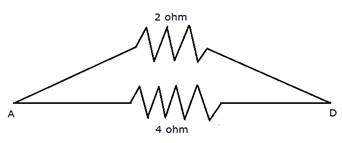These two resistors are in parallel combination , so when we solve these two we will get ,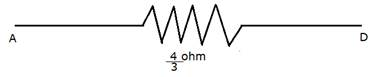This is the resultant resistance.Question 7.Find the resistance acrossB and D.Answer:In order to find the total resistance, first we need to memorize the rule which we have learned which is , THE POINTS WHOSE ACROSS WE HAVE TO EVALUATE THE TOTAL RESISTANCE ARE THE GIVEN POINTS AND WE CONSIDER OR IMAGINE THAT A BATTERY IS CONNECTED TO THE CIRCUIT THROUGH THESE TWO POINTS ONLY .B and D are the given points in this part and the remaining are the simple points to deal with (that is A and C).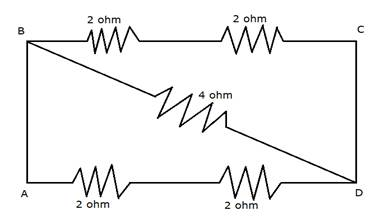The resistors in the line BC are in series as well as the resistors in the line AD are also in series with each other, so by solving we get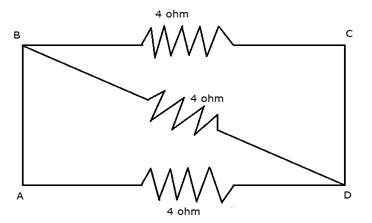Merge the point A into point B and also merge the point C into point D, all three resistors are parallel to each other, so,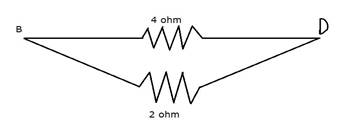These two resistors are parallel to each other, so by solving them we get,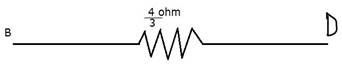Thus, it is the resultant or total resistance.Question 8.Explain the two different ways of harnessing energy from the ocean.Answer:Basically there are three ways of harnessing energy from the oceans and seas, but we are today going to discuss only two,Which are :-1. OCEAN THERMAL ENERGY :-The water at the surface of the sea or ocean which, has considerable depth or deepness, is heated by the Sun while as we go deeper and deeper in the ocean or sea the upcoming sections become relatively colder than the previous one. This difference in temperature is used to obtain energy in ocean-thermal-energy conversion plants. These plants can operate if the temperature difference between the water at the surface and water at depths up to 2 km is 293 K (20°C) or more. The warm surface-water is used to boil a volatile liquid like ammonia. The vapours of the liquid are then used to run the turbine of generator. The cold water from the depth of the ocean is pumped up and condense vapour again to liquid.2. OCEAN TIDAL ENERGY :-Due to the gravitational pull of mainly the moon on the spinning earth, the level of water in the sea rises and falls. This phenomenon is called high and low tides and the difference in sea-levels gives us tidal energy by the same method as we saw in hydroelectric power plant , but in this method the gravitational energy by moon gives potential energy as well as kinetic energy to the water thereby there is formation of tides or waves. Tidal energy is harnessed by constructing a dam across a narrow opening to the sea. A turbine fixed at the opening of the dam converts tidal energy to electricity.There is also Ocean wave energy.Question 9.Five resistors of resistance ‘R’ are connected such that they form a letter ‘A’. Find the effective resistance across the free ends.Answer:The simple resistors must be identified and after that they must be first solved and when they are solved look for again the simple resistors pattern solve till you end up with only one resistor.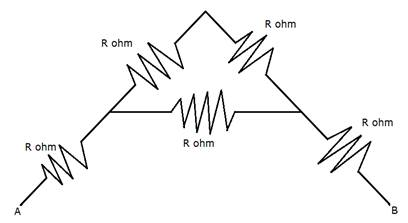We will solve diagram by diagram,First we will add the two top most resistors because they are in series, we get ,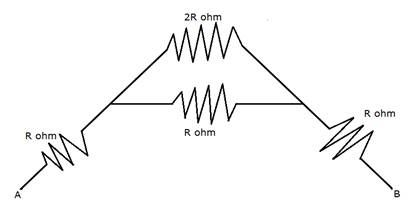Now the two topmost resistors are parallel to each other because their free ends are attached to same points if we look it as wire.We get,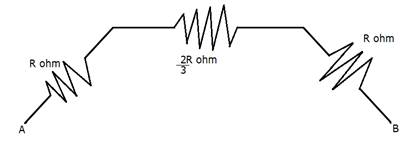Now these three are in series with each other, so we will add them simply like we are adding integers.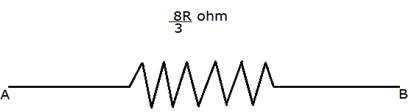We will get,Therefore, the total or the resultant resistance is = 8R/3 ohm.

PDF FILE TO YOUR EMAIL IMMEDIATELY PURCHASE NOTES & PAPER SOLUTION. @ Rs. 50/- each (GST extra)

HINDI ENTIRE PAPER SOLUTION

MARATHI PAPER SOLUTION

SSC MATHS I PAPER SOLUTION

SSC MATHS II PAPER SOLUTION

SSC SCIENCE I PAPER SOLUTION

SSC SCIENCE II PAPER SOLUTION

SSC ENGLISH PAPER SOLUTION

SSC & HSC ENGLISH WRITING SKILL

HSC ACCOUNTS NOTES

HSC OCM NOTES

HSC ECONOMICS NOTES

HSC SECRETARIAL PRACTICE NOTES

2019 Board Paper Solution

HSC ENGLISH SET A 2019 21st February, 2019

HSC ENGLISH SET B 2019 21st February, 2019

HSC ENGLISH SET C 2019 21st February, 2019

HSC ENGLISH SET D 2019 21st February, 2019

SECRETARIAL PRACTICE (S.P) 2019 25th February, 2019

HSC XII PHYSICS 2019 25th February, 2019

CHEMISTRY XII HSC SOLUTION 27th, February, 2019

OCM PAPER SOLUTION 2019 27th, February, 2019

HSC MATHS PAPER SOLUTION COMMERCE, 2nd March, 2019

HSC MATHS PAPER SOLUTION SCIENCE 2nd, March, 2019

SSC ENGLISH STD 10 5TH MARCH, 2019.

HSC XII ACCOUNTS 2019 6th March, 2019

HSC XII BIOLOGY 2019 6TH March, 2019

HSC XII ECONOMICS 9Th March 2019

SSC Maths I March 2019 Solution 10th Standard11th, March, 2019

SSC MATHS II MARCH 2019 SOLUTION 10TH STD.13th March, 2019

SSC SCIENCE I MARCH 2019 SOLUTION 10TH STD. 15th March, 2019.

SSC SCIENCE II MARCH 2019 SOLUTION 10TH STD. 18th March, 2019.

SSC SOCIAL SCIENCE I MARCH 2019 SOLUTION20th March, 2019

SSC SOCIAL SCIENCE II MARCH 2019 SOLUTION, 22nd March, 2019

XII CBSE - BOARD - MARCH - 2019 ENGLISH - QP + SOLUTIONS, 2nd March, 2019

HSCMaharashtraBoardPapers2020

(Std 12th English Medium)

HSC ECONOMICS MARCH 2020

HSC OCM MARCH 2020

HSC ACCOUNTS MARCH 2020

HSC S.P. MARCH 2020

HSC ENGLISH MARCH 2020

HSC HINDI MARCH 2020

HSC MARATHI MARCH 2020

HSC MATHS MARCH 2020

SSCMaharashtraBoardPapers2020

(Std 10th English Medium)

English MARCH 2020

HindI MARCH 2020

Hindi (Composite) MARCH 2020

Marathi MARCH 2020

Mathematics (Paper 1) MARCH 2020

Mathematics (Paper 2) MARCH 2020

Sanskrit MARCH 2020